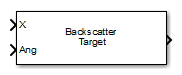## Library

Environment and Target

`phasedenvlib`

•## Description

The Backscatter Radar Target block models the monostatic case of reflection of nonpolarized electromagnetic signals from a radar target. Target model includes all four Swerling target fluctuation models and non-fluctuating model. You can model several targets simultaneously by specifying multiple radar cross-section matrices.

## Parameters

Azimuth angles (deg)

Azimuth angles used to define the angular coordinates of the RCS pattern (m^2) parameter. Specify azimuth angles as a length P vector. Units are degrees. P must be greater than two. This parameter determines the incident azimuthal arrival angle of any element of the cross-section patterns.

Elevation angles (deg)

Elevation angles used to define the angular coordinates of the RCS pattern (m^2) parameter. Specify elevation angles as a length Q vector. Units are degrees. Q must be greater than two. This parameter determines the incident elevation arrival angle of any element of the cross-section patterns.

RCS pattern (m^2)

Radar cross-section pattern, specified as a Q-by-P real-valued matrix or a Q-by-P-by-M real-valued array.

• Q is the length of the vector in the Elevation angles (deg) parameter.

• P is the length of the vector in the Azimuth angles (deg) parameter.

• M is the number of target patterns. The number of patterns corresponds to the number of signals passed into the input port `X`. You can, however, use a single pattern to model multiple signals reflecting from a single target.

You can, however, use a single pattern to model multiple signals reflecting from a single target. Pattern units are square-meters.

Pattern units are square-meters.

Fluctuation model

Specify the statistical model of the target as either `Nonfluctuating`, `Swerling1`, `Swerling2`, `Swerling3`, or `Swerling4`. When you set this parameter to a value other than `Nonfluctuating`, you then set radar cross-sections parameters using the `Update` input port.

Signal Propagation speed (m/s)

Specify the propagation speed of the signal, in meters per second, as a positive scalar. You can use the function `physconst` to specify the speed of light.

Operating frequency (Hz)

Specify the carrier frequency of the signal that reflects from the target, as a positive scalar in hertz.

Simulate using

Block simulation method, specified as `Interpreted Execution` or ```Code Generation```. If you want your block to use the MATLAB® interpreter, choose `Interpreted Execution`. If you want your block to run as compiled code, choose `Code Generation`. Compiled code requires time to compile but usually runs faster.

Interpreted execution is useful when you are developing and tuning a model. The block runs the underlying System object™ in MATLAB. You can change and execute your model quickly. When you are satisfied with your results, you can then run the block using ```Code Generation```. Long simulations run faster than they would in interpreted execution. You can run repeated executions without recompiling. However, if you change any block parameters, then the block automatically recompiles before execution.

When setting this parameter, you must take into account the overall model simulation mode. The table shows how the Simulate using parameter interacts with the overall simulation mode.

When the Simulink® model is in `Accelerator` mode, the block mode specified using Simulate using overrides the simulation mode.

Acceleration Modes

 Block Simulation Simulation Behavior `Normal` `Accelerator` `Rapid Accelerator` `Interpreted Execution` The block executes using the MATLAB interpreter. The block executes using the MATLAB interpreter. Creates a standalone executable from the model. `Code Generation` The block is compiled. All blocks in the model are compiled.

## Ports

Note

The block input and output ports correspond to the input and output parameters described in the `step` method of the underlying System object. See link at the bottom of this page.

PortDescriptionSupported Data Types
`X`

Input signals.

The size of the first dimension of the input matrix can vary to simulate a changing signal length. A size change can occur, for example, in the case of a pulse waveform with variable pulse repetition frequency.

Double-precision floating point
`Ang`Incident angleDouble-precision floating point
`Update`Update RCS at block execution.Double-precision floating point
`Out`Scattered signalDouble-precision floating point

## Version History

Introduced in R2016a